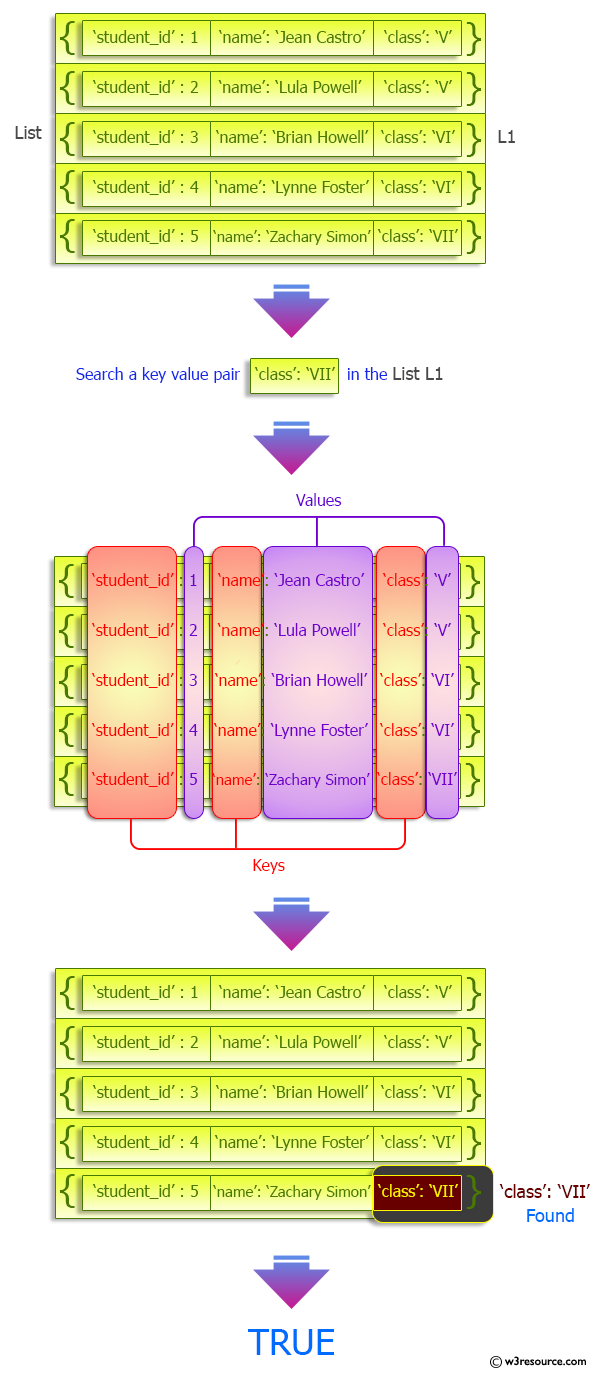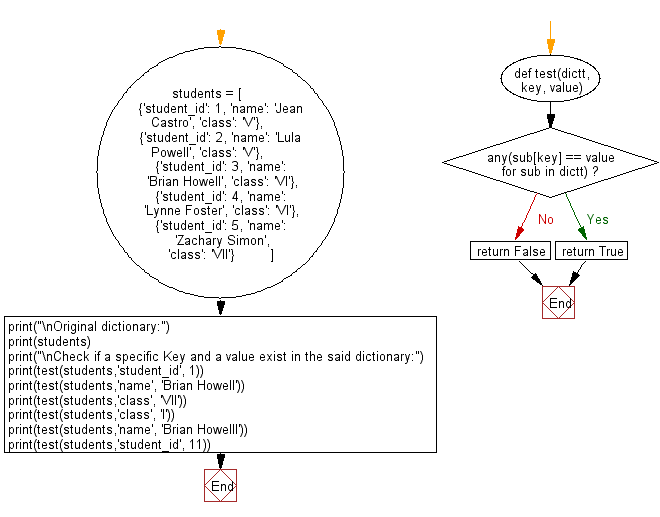﻿ Python: Check if a specific Key and a value exist in a dictionary - w3resource# Python: Check if a specific Key and a value exist in a dictionary

## Python dictionary: Exercise-66 with Solution

Write a Python program to check if a specific Key and a value exist in a dictionary.

Sample Solution:

Python Code:

``````def test(dictt, key, value):
if any(sub[key] == value for sub in dictt):
return True
return False

students = [
{'student_id': 1, 'name': 'Jean Castro', 'class': 'V'},
{'student_id': 2, 'name': 'Lula Powell', 'class': 'V'},
{'student_id': 3, 'name': 'Brian Howell', 'class': 'VI'},
{'student_id': 4, 'name': 'Lynne Foster', 'class': 'VI'},
{'student_id': 5, 'name': 'Zachary Simon', 'class': 'VII'}
]

print("\nOriginal dictionary:")
print(students)
print("\nCheck if a specific Key and a value exist in the said dictionary:")
print(test(students,'student_id', 1))
print(test(students,'name', 'Brian Howell'))
print(test(students,'class', 'VII'))
print(test(students,'class', 'I'))
print(test(students,'name', 'Brian Howelll'))
print(test(students,'student_id', 11))
```
```

Sample Output:

```Original dictionary:
[{'student_id': 1, 'name': 'Jean Castro', 'class': 'V'}, {'student_id': 2, 'name': 'Lula Powell', 'class': 'V'}, {'student_id': 3, 'name': 'Brian Howell', 'class': 'VI'}, {'student_id': 4, 'name': 'Lynne Foster', 'class': 'VI'}, {'student_id': 5, 'name': 'Zachary Simon', 'class': 'VII'}]

Check if a specific Key and a value exist in the said dictionary:
True
True
True
False
False
False
```

Pictorial Presentation:Flowchart:## Visualize Python code execution:

The following tool visualize what the computer is doing step-by-step as it executes the said program:

Python Code Editor:

Have another way to solve this solution? Contribute your code (and comments) through Disqus.

What is the difficulty level of this exercise?

Test your Programming skills with w3resource's quiz.

﻿

## Python: Tips of the Day

Invokes the provided function after ms milliseconds:

Example:

```from time import sleep

def tips_delay(fn, ms, *args):
sleep(ms / 1000)
return fn(*args)
print(tips_delay(
lambda x: print(x),
1000,
'w3r'
))
```

Output:

```w3r
None
```